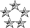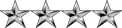## What is Permutations & Combinations?

Mathematics for Computing
NeoPosts: 2642
Joined: Wed Jul 15, 2009 2:07 am
Location: Colombo

### What is Permutations & Combinations?

Since there are lots of explanations in the internet, I'll explain them briefly and go to few examples.

Permutation: Permutation means arrangement of things. The word arrangement is used, if the order of things is considered.
ORDER IS IMPORTANT
පිලිවෙලකට අනුව සැකසිය හැකි ආකාර

Combination: Combination means selection of things. The word selection is used, when the order of things has no importance.
ORDER IS NOT IMPORTANT
පිලිවෙලක් නැතුව තොරාගත හැකි ආකාර. පිලිවෙලක් නැති නිසා එකම පදය නැවත නැවතත් ලියනු නොලැබෙ.

The only things you need to remember are the formulas.

Permutation Formula:
nPr = n! / (n - r)! Where r <= n
Read as, we have n items and want to pick k in a certain order.

Combination Formula:
nCr = n! / ( r! x (n - r)! ) Where r <= n

Read as, we have n items and want to pick k number of items at a time.

If you are not familiar with n! (Factorial n), have a look at What is factorial?.
n! = 1 x 2 x 3 x ....... (n - 2) x (n - 1) x n

Though these might look quite strange, believe me these are very simple to use.

Let's take an example:

Say there are 5 letters, A B C D E. You are asked to pick 3 letters in order.
Here the n = 5 and k =3.
5P3 = 5! / (5 - 3)! = 5! / 2! = (5 x 4 x 3 x 2 x 1) / (2 x 1) = 5 x 4 x 3 = 60
So there are 60 ways to select 3 letters from a set of 5 letters in a particular order.
Here ABC and CAB are counted as 2 since the order is important.

If you are asked to pick 3 letters without considering the order (so that a combination) here is how we can do it.

5C3 = 5! / 3! x (5 - 3)! = (5 x 4 x 3 x 2 x 1) / (3 x 2 x 1) x (2 x 1) = 5 x 2 = 10
So there are 10 ways to select 3 letters from a set of 5 letters without an order.
Here ABC and CAB are counted as 1 since the order is NOT considered.

Note that we are talking about n items in a particular set here. If you were asked to chose items repeatedly on the same set, then you will need to multiply the result. For example, you are asked to select 1 letter from the set A and B. Then again asked to select 1 letter from A and B (Not considering that 1 is already select before). Finally the same process to chose 1 letter from A and B. So altogether we were asked 3 times to chose one letter each time on the same set then this is 3 combinations. Don't try to apply this on the same formula.
2C1 X 2C1 X 2C1 = 8

I guess you now have a simple idea on Permutations & Combinations now. Lets answer your posts now.
Nipuna
ModeratorPosts: 2729
Joined: Mon Jan 04, 2010 8:02 pm
Location: Deraniyagala,SRI LANKA

### Re: What is Permutations & Combinations?

Cool ManI knew Those Formulas. But Any Tutorial I found Newer Gave Me such Clear Idea.

Thanks a Lot Friend